# Icon making with ggplot2 and magickIcon
noun
A person or thing regarded as a representative symbol or as worthy of veneration.
A symbol or graphic representation on a screen of a program, option, or window.
from Oxford English Dictionaries

Fontawesome and the noun project along with other icon provides produce and distribute beautiful icons for free use. But sometimes, I would like to alter or create my own tiny 16x16 or 32x32 pixel wide icons without having to learn inkscape or any other svg editor. The figure should have one/two colors on a transparent background.

So first I will try to make an icon of a normal distribution using ggplot2. We start with a typical normal distribution on the basic ggplot2 theme.

library(ggplot2)
p <- ggplot(data.frame(x = c(-2, 2)), aes(x)) +
stat_function(fun = dnorm)
p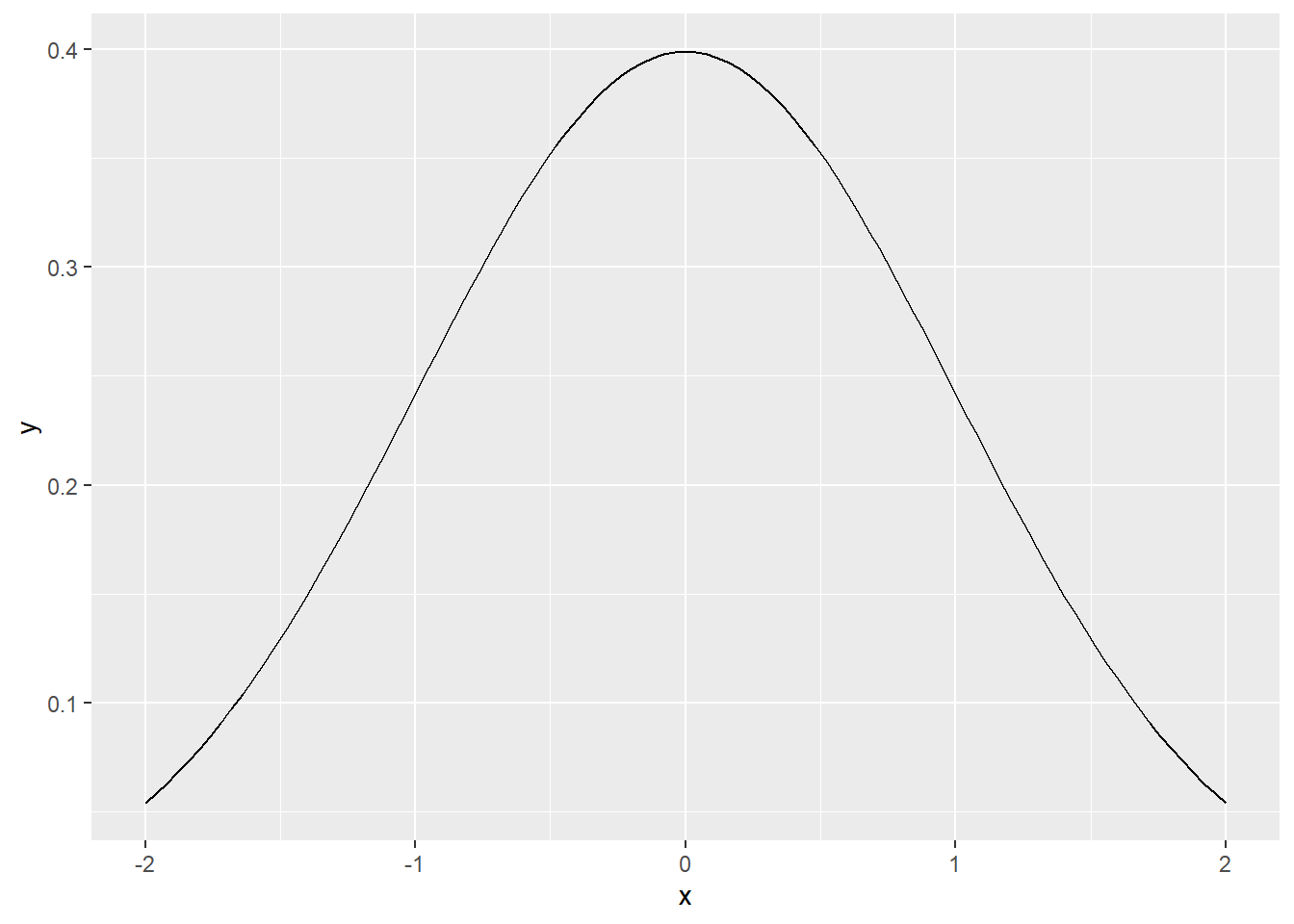In this case, we want a white icon so the curve should be white.

p <- ggplot(data.frame(x = c(-2, 2)), aes(x)) +
stat_function(fun = dnorm, color = "white")
p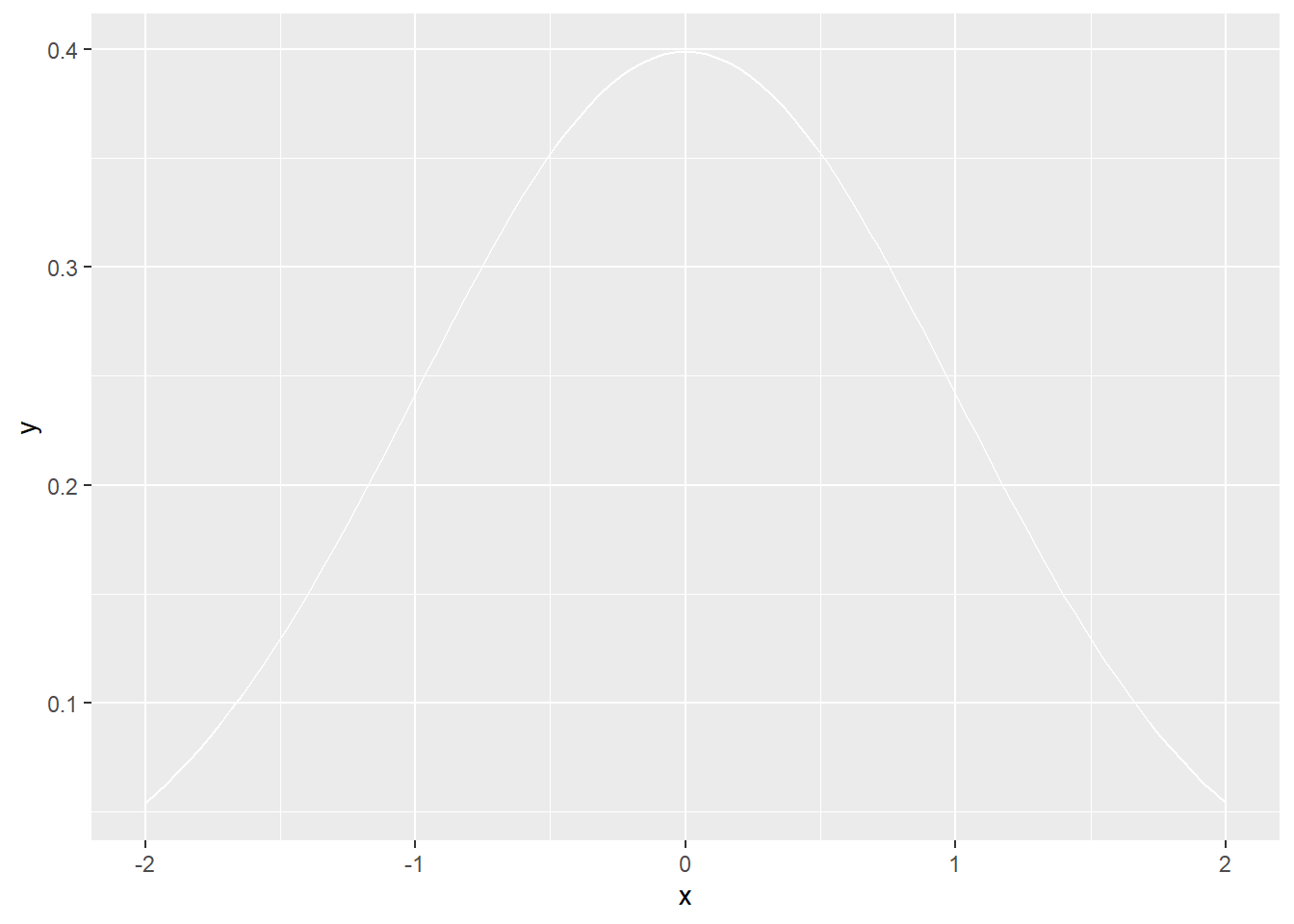p + ggtitle("Icon")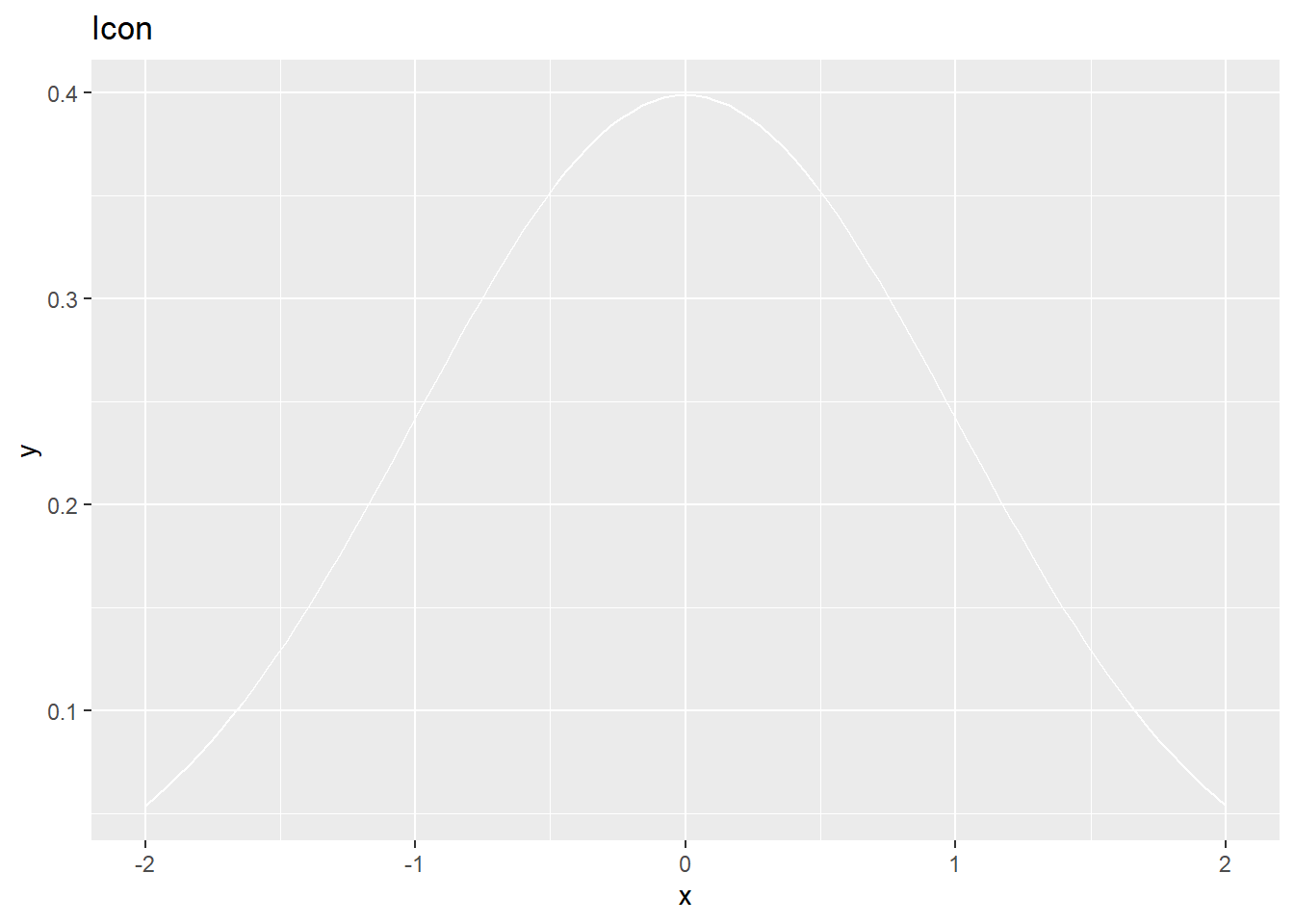To make the background transparent, we can edit the panel.background and plot.background elements in theme.

p + ggtitle("Icon") +
theme(panel.background = element_rect(fill="transparent",colour=NA),
plot.background = element_rect(fill="transparent",colour=NA))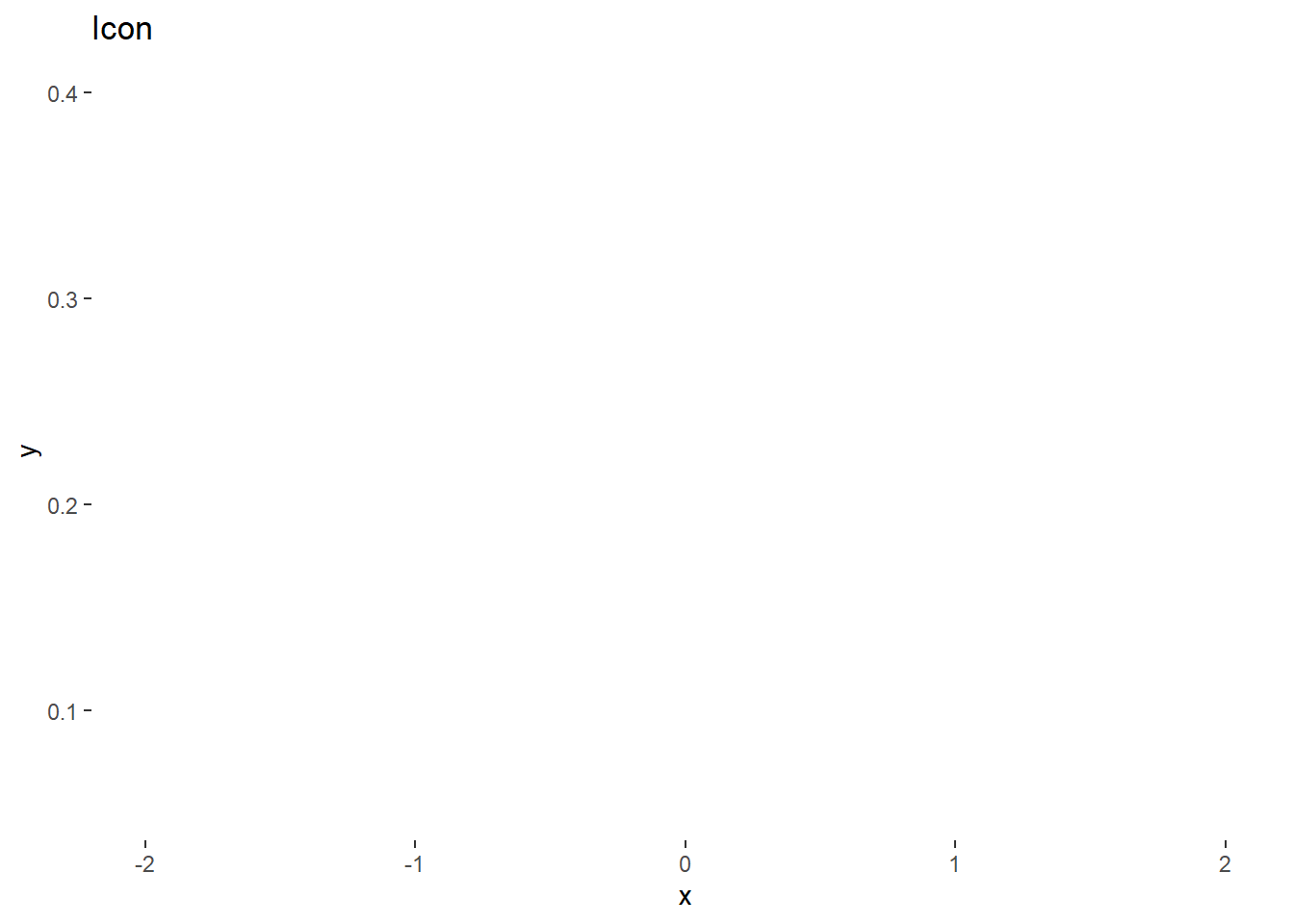This doesn’t look very informative so we can just change one of the backgrounds temporarily so we can see the different elements.

p + ggtitle("Icon") +
theme(panel.background = element_rect(fill="transparent",colour=NA),
plot.background = element_rect(fill="grey",colour=NA))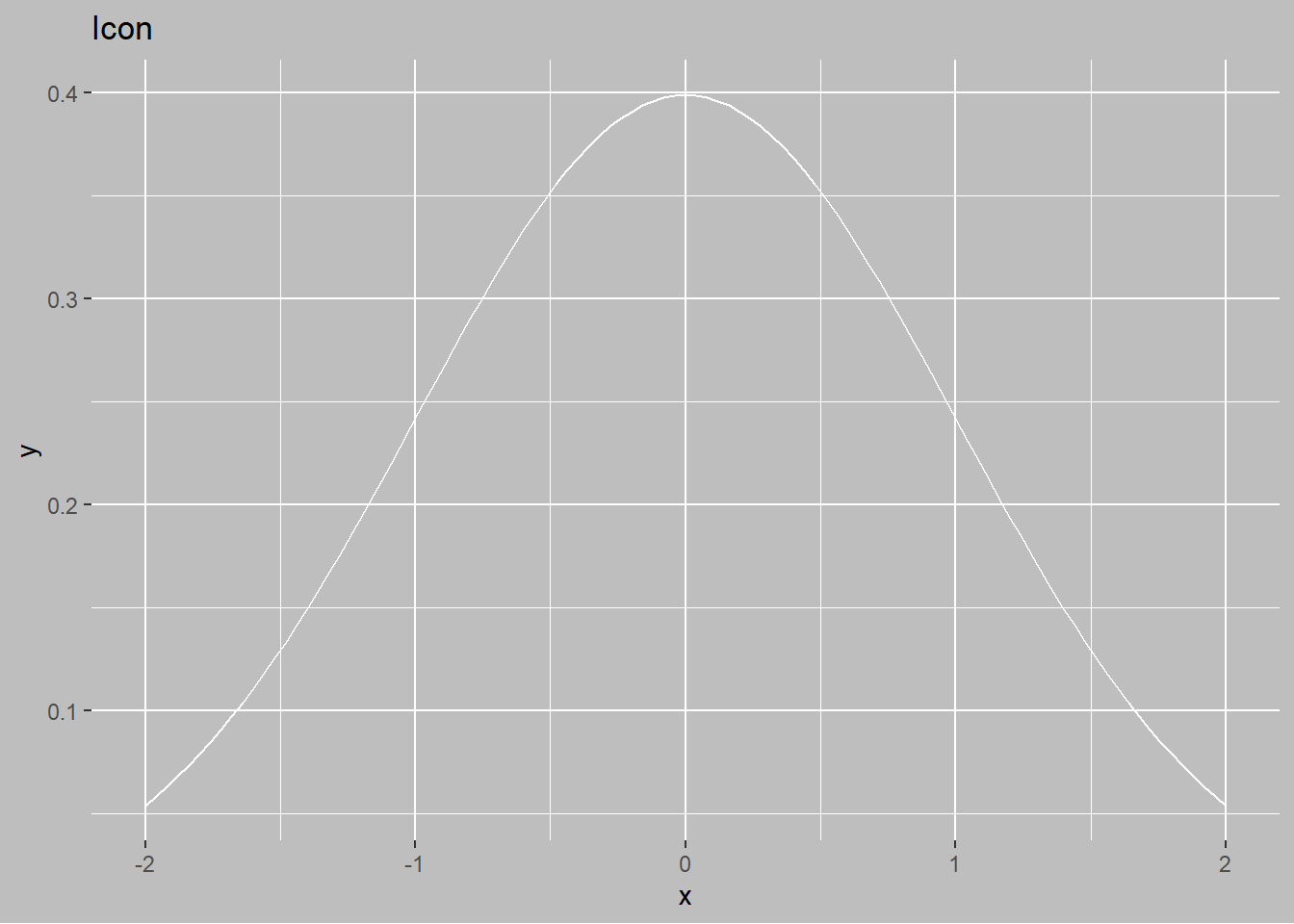The elements outside the actual plot is controlled by text, line, and rect (as explained here). So we can set all three to white as well.

p + ggtitle("Icon") +
theme(panel.background = element_rect(fill="transparent",colour=NA),
plot.background = element_rect(fill="grey",colour=NA),
text = element_text(color = "white"),
line = element_line(color = "white"),
rect = element_rect(fill = "transparent", colour = NA))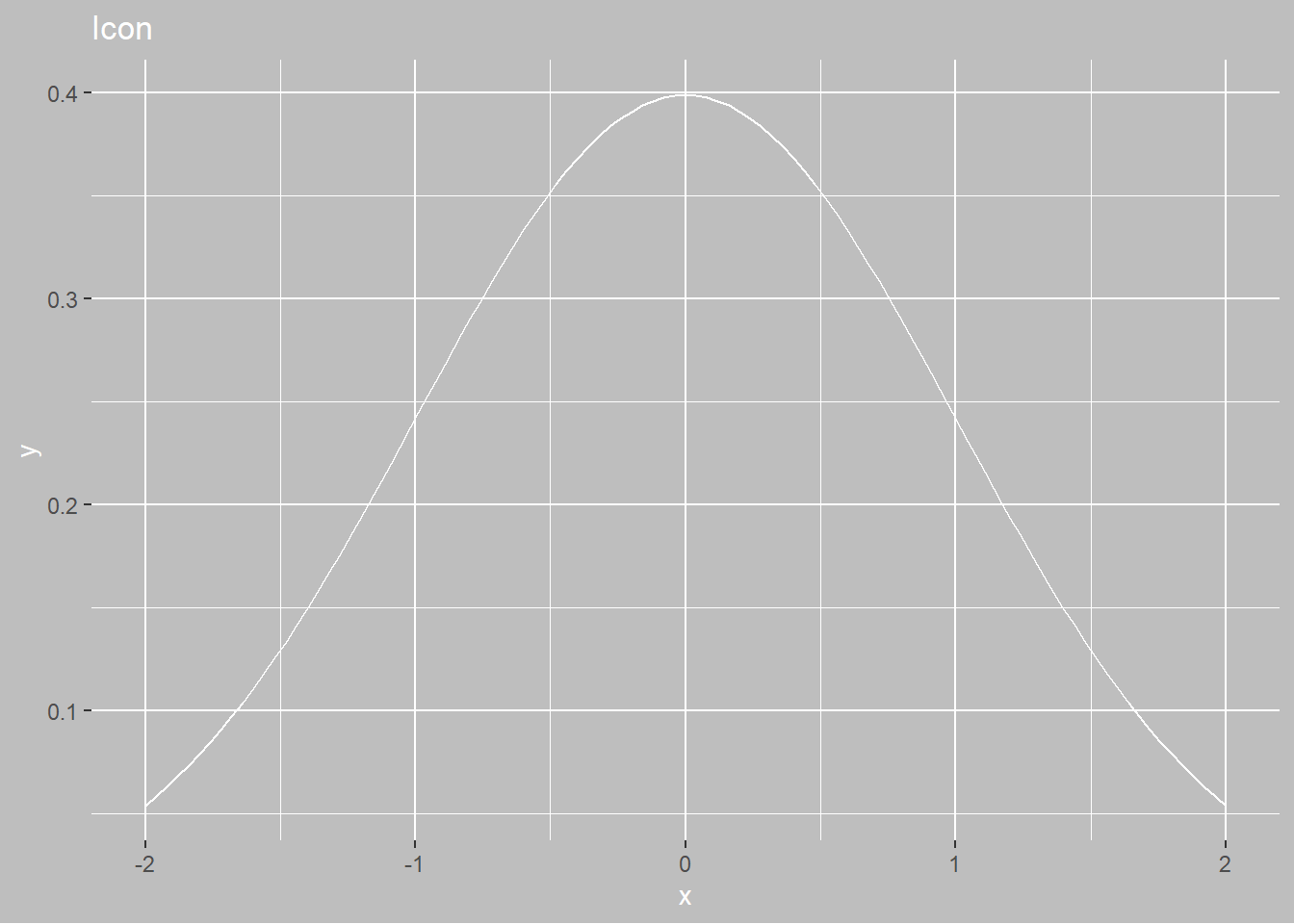The axis tick marks and grid lines would not be extraneous in an icon and can be removed as well.

p + ggtitle("Icon") +
theme(panel.background = element_rect(fill="transparent",colour=NA),
plot.background = element_rect(fill="grey",colour=NA),
text = element_text(color = "white"),
line = element_line(color = "white"),
rect = element_rect(fill = "transparent", colour = NA),
axis.text = element_blank(),
axis.ticks = element_blank(), panel.grid = element_blank())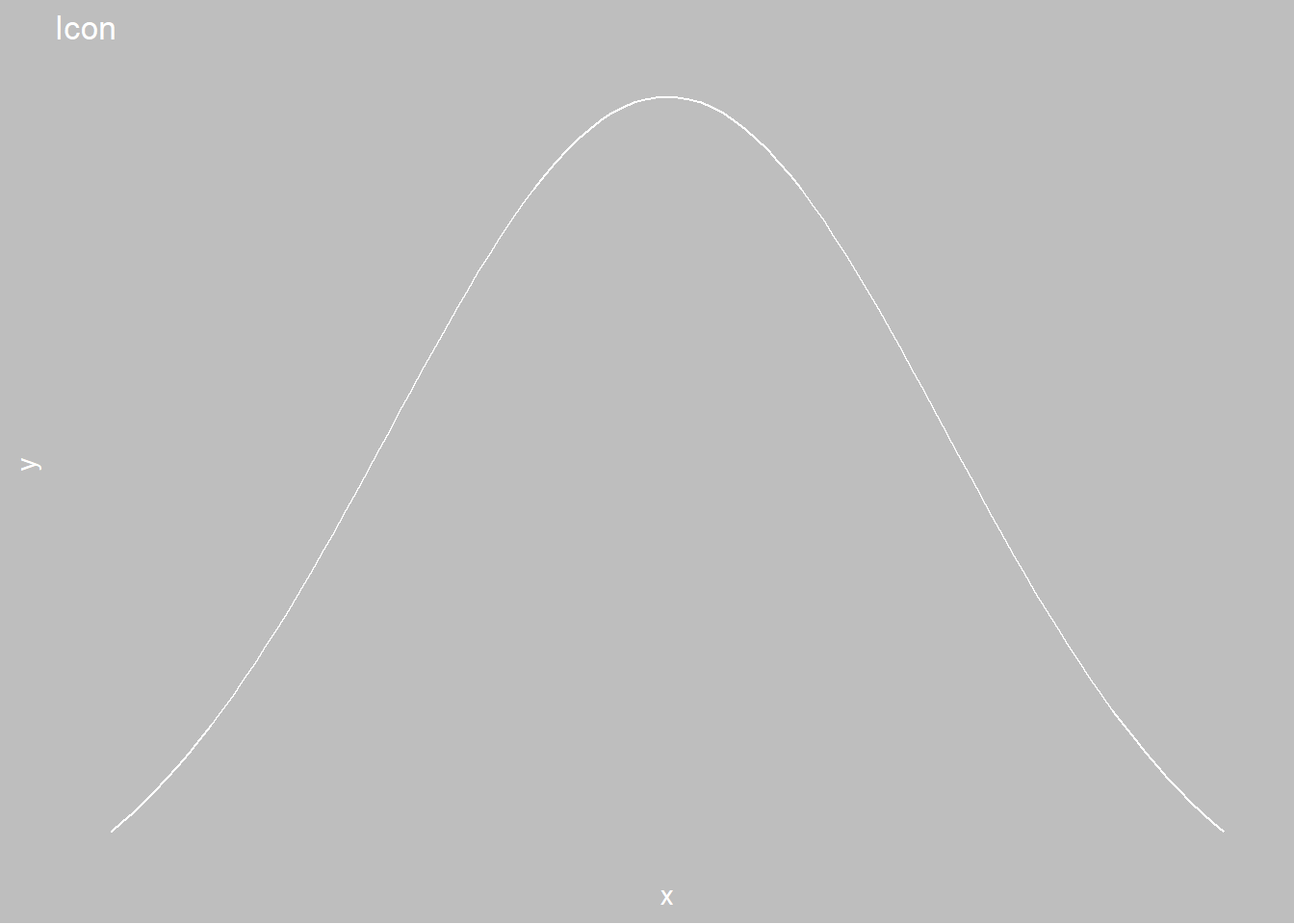We can add back the axis lines with axis.line.

p + ggtitle("Icon") +
theme(panel.background = element_rect(fill="transparent",colour=NA),
plot.background = element_rect(fill="grey",colour=NA),
text = element_text(color = "white"),
line = element_line(color = "white"),
rect = element_rect(fill = "transparent", colour = NA),
axis.text = element_blank(),
axis.ticks = element_blank(), panel.grid = element_blank(),
axis.line = element_line(color = "white"))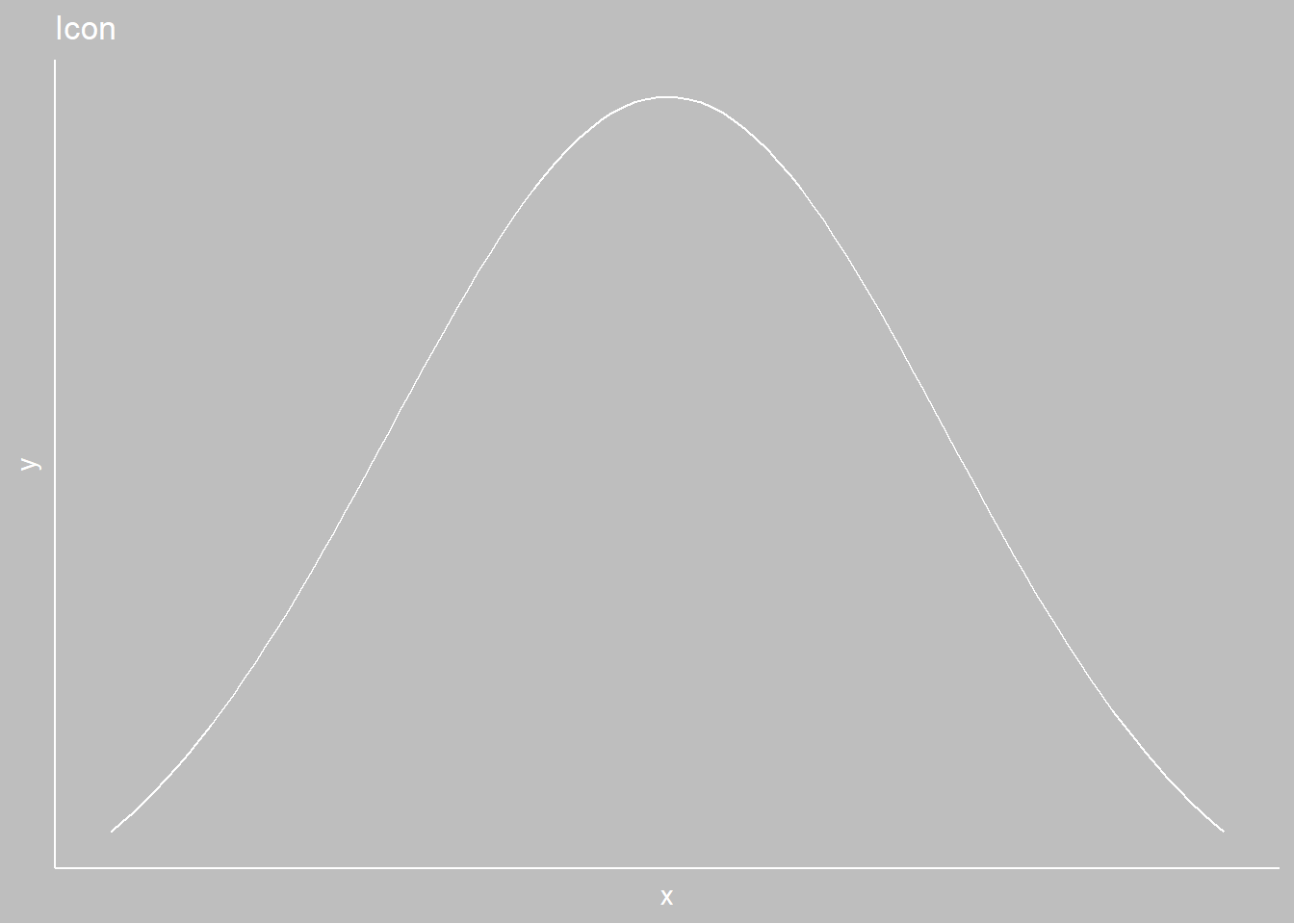For the real plot, we want the background to be transparent rather than gray.

icon_plot <- p + ggtitle("Icon") +
theme(panel.background = element_rect(fill="transparent",colour=NA),
plot.background = element_rect(fill="transparent",colour=NA),
text = element_text(color = "white"),
line = element_line(color = "white"),
rect = element_rect(fill = "transparent", colour = NA),
axis.text = element_blank(),
axis.ticks = element_blank(), panel.grid = element_blank(),
axis.line = element_line(color = "white"))

We can save this resulting plot as both svg and png using ggsave. As ggsave uses width and height in inches/centimeters but we would like to produce images of pixel size, we can use the dpi parameter to avoid doing any math.

These transparent plots can also work on colored infographics as abstract figures but convey much more detail. since ggplot2 allows for detailed control of the plot, changing text and line sizes can generate all kinds of

A 72x72 pixel svg.

ggsave(filename = "icon_72px.svg", icon_plot, dpi=72, width = 1, height = 1)
## Warning: package 'gdtools' was built under R version 3.6.2

A 72x72 pixel png icon. The important argument here is passing the bg = "transparent" argument into the png device.

ggsave(filename = "icon_72px.png", icon_plot, dpi=72, width = 1, height = 1, bg = "transparent")

To actually show the white icon on the page, I’m manually adding a background color.

<img src="icon_72px.png" alt="72x icon with blue background" style="background-color:#9ecff7;"/>On the other hand, if you already have an image you want to convert to an icon, we can use the magick package to use imagemagick for image processing and scaling.

library(magick)
## Warning: package 'magick' was built under R version 3.6.2
## Linking to ImageMagick 6.9.9.14
## Enabled features: cairo, freetype, fftw, ghostscript, lcms, pango, rsvg, webp
## Disabled features: fontconfig, x11

We will use the example tiger image from the magick vignette.

tiger <- image_read_svg('http://jeroen.github.io/images/tiger.svg', width = 400)
print(tiger)
## # A tibble: 1 x 7
##   format width height colorspace matte filesize density
##   <chr>  <int>  <int> <chr>      <lgl>    <int> <chr>
## 1 PNG      400    400 sRGB       TRUE         0 72x72First, we convert color to two colors only with image_convert.

tiger %>% image_convert(type = "bilevel")Since the background is black, and we would like it transparent, we can flip the black and white.

tiger %>% image_convert(type = "bilevel") %>% image_negate()And lastly, we can scale to pixel size with image_scale.

tiger %>% image_convert(type = "bilevel") %>% image_negate() %>% image_scale("72x")imagemagick has much better capabilities and I feel that saving a full-size plot with ggsave and then scaling with magick will create better icons than using ggsave and specifying dpi as above.